Courses

# Test: Oxidation Number (Redox Reaction)

## 19 Questions MCQ Test Chemistry for JEE | Test: Oxidation Number (Redox Reaction)

Description
This mock test of Test: Oxidation Number (Redox Reaction) for JEE helps you for every JEE entrance exam. This contains 19 Multiple Choice Questions for JEE Test: Oxidation Number (Redox Reaction) (mcq) to study with solutions a complete question bank. The solved questions answers in this Test: Oxidation Number (Redox Reaction) quiz give you a good mix of easy questions and tough questions. JEE students definitely take this Test: Oxidation Number (Redox Reaction) exercise for a better result in the exam. You can find other Test: Oxidation Number (Redox Reaction) extra questions, long questions & short questions for JEE on EduRev as well by searching above.
QUESTION: 1

### Direction (Q. Nos. 1-12) This section contains 12 multiple-choice questions. Each question has four choices (a), (b), (c) and (d), out of which ONLY ONE option is correct. Consider the following reactions. I. Zn + dil. H2So4 → ZnSO4 + H2 II. Zn + conc. H2SO4 → ZnSO4 + SO4 + H2O III. Zn + HNO3 → Zn(NO3)2 + H2O + NH4NO3 Q.  Oxidation number of H changes in:

Solution: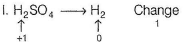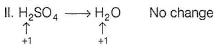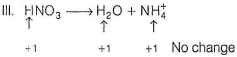QUESTION: 2

### In which case change of oxidation number of V is maximum?

Solution: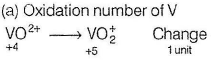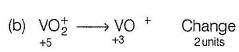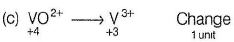QUESTION: 3

### In which of the following set of compounds oxidation number of oxygen is not (- 2)?

Solution: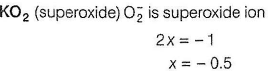OF2 Fluorine is most electronegative atom with oxidation number = - 1
x - 2 = 0
x = + 2
H2O2   2 + 2x = 0 ⇒ x = - 1

QUESTION: 4

Which is chlorate (I) ion?

Solution:
• ClO3: A very reactive inorganic anion.
• The term chlorate can also be used to describe any compound containing the chlorate ion, normally chlorate salts.
• Example: Potassium chlorate, KClO3
QUESTION: 5

Which of the following is not an example of redox reaction?

Solution:

a) BaCl2 + H2SO4 → BaSO4 + 2HCl is not a redox reaction, as there is no change in the oxidation state of any element.
It is an example of double displacement reactions.

QUESTION: 6

Which of the following atom has been assigned only single oxidation number?

Solution:
• Fluorine is the most electronegative element. It can gain one electron.​
Thus, Oxidation number = -1
F+ e- → F-
• Due to very high (IE), it cannot lose electrons.

(a) H- (-1), (H+ (+1)
(b) O -2 in oxide, -1 in peroxide, and +2 in OF2
(c) +3 in N2O3, -3 in NH3, +3 in HNO2, +5 in HNO3, + 1 in N2O

QUESTION: 7

The oxidation number of P in Ba(H2PO2)2, Ba(H2PO3)2 and Ba(H2PO4)2 are respectively

Solution: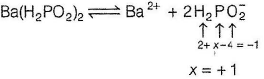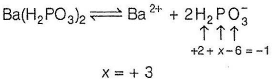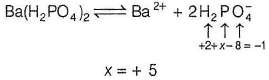QUESTION: 8

the oxidation number of sulphur in S8,S2F2,H2S respectively are

Solution:

(i) Oxidation state of element in its free state is zero.

(ii) Sum of oxidation states of all atoms in compound is zero.

O.N of S in S8=0;    O.N of S in S2F2=+1

O.N of S in H2S=-2

QUESTION: 9

In which of the following reactions oxidation number of chromium has been affected?

Solution: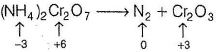Oxidation number of Cr changes from +6 to +3.

QUESTION: 10

Prussian blue is represented by KFex[Fe(CN)6]. Value of x is

Solution: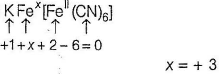QUESTION: 11

In the following reaction,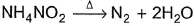, oxidation number of

Solution:
QUESTION: 12

Select the set of compounds having fractional oxidation number in one or more atoms.

Solution: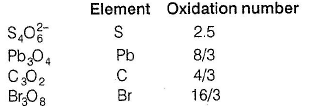*Multiple options can be correct
QUESTION: 13

Direction (Q. Nos. 13 and  14) This section contains 2 multiple choice questions. Each question has four choices (a), (b), (c) and (d), out of which ONE or  MORE THANT ONE  is correct.

Q. Which of the following species has/have oxidation number of the metal as + 6 ?

Solution: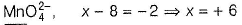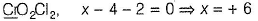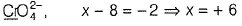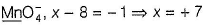QUESTION: 14

Oxidation numbers of P in PO4−3, of S in SO42− and that of Cr in Cr2O72− are respectively,

Solution:

The correct answer is option A
(I) xPO43−​ ⇒ x + 4 × (−2) = −3
⇒x = −3 + 8 = +5
⇒x = +5
Oxidation number of P = +5
(II) xSO42−​ ⇒ x + 4 × (−2) = −2
⇒x = −2 + 8
⇒x = +6
Oxidation number of S=+6
(III) xCr2​O72− ​    ⇒2x + 7 × (−2) = −2
⇒2x =−2+14
⇒2x=12
⇒x= 12/2​ = +6

*Multiple options can be correct
QUESTION: 15

Direction (Q. Nos. 15-16) This section contains  a paragraph, wach describing theory, experiments, data etc. three Questions related to paragraph have been given.Each question have only one correct answer among the four given options (a),(b),(c),(d)

The complex [Fe(H2O)5NO]2+ is formed in the ring-test for nitrate ion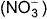when freshly prepared FeSO4 solution is added to aqueous solution of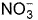followed by the addition of conc. H2SO4. NO exists as NO(nitrosyl).

Q.Oxidation number of the Fe in the ring is

Solution:

Let, oxidation number of Fe = x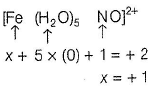*Multiple options can be correct
QUESTION: 16

The complex [Fe(H2O)5NO]2+ is formed in the ring-test for nitrate ionwhen freshly prepared FeSO4 solution is added to aqueous solution offollowed by the addition of conc. H2SO4. NO exists as NO(nitrosyl).

Q. Magnetic moment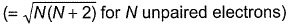of Fe in the ring is

Solution:

Fe2+ is added asFeSO4
Fe+ is formed by charge transfer from NO to Fe2+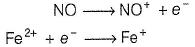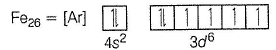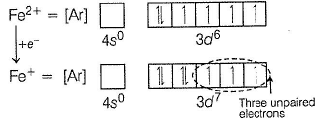Fe+ has three unpaired electrons (N).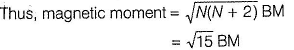QUESTION: 17

Direction (Q. Nos. 17) Choice the correct combination of elements and column I and coloumn II are given as option (a), (b), (c) and (d), out of which ONE option is correct.

Match the compounds/ions having underlined atoms of different oxidation number (in Column I) with values (in Column II).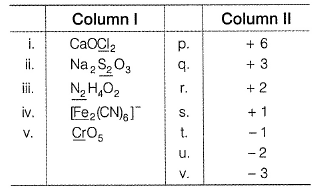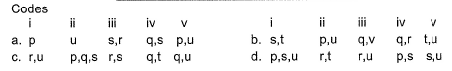Solution:

(i) CaOCI2 has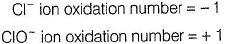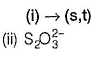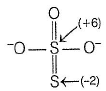Oxidation number = + 6
Oxidation number = - 2
(ii) → (p,u)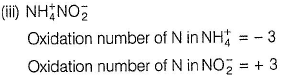(iii) → (q.v)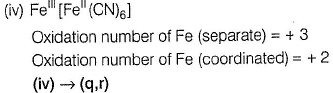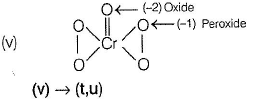*Answer can only contain numeric values
QUESTION: 18

Direction (Q. Nos. 18 and 19) This section contains 2 questions. when worked out will result in an integer from 0 to 9 (both inclusive)

Q.   S2O32- has two types of sulphur atoms. What is the difference in the oxidation states of two types of sulphur atoms?

Solution: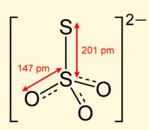The two sulfur atoms of thiosulfate exist in the oxidation state of sulfate (+6) and sulfide (−2) and do not change their respective oxidation states upon disproportionation.

So difference in the oxidation states of two types of sulphur atoms will be

= (+6)- (-2)

= +8

QUESTION: 19

The difference in the oxidation number of the two types of sulphur atoms in Na2S4O6 is _________. [HTJEE2011]

Solution: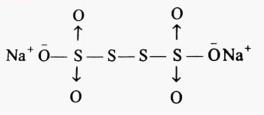the difference of oxidation states of two S is 5−0 = 5